ExetoolsKeygenme (Easy?)
 Register Forum Rules FAQ Calendar Mark Forums Read

 Notices HTTP connection will be closed soon. Please visit eXeTools with HTTPS in the future. https://forum.exetools.com This is the ONLY ONE domain that we use. Follow @exetools on Twitter and send me a message, I will choose whether to send the invitation code. Any password problems please mailto: 883600(at)qq(dot)com

#1
 dilaFriend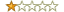Join Date: Jan 2010 Posts: 60 Rept. Given: 12 Rept. Rcvd 32 Times in 14 Posts Thanks Given: 35 Thanks Rcvd at 74 Times in 20 Posts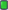Keygenme (Easy?)

Hi, I made a keygenme for you all to try. I am very interested to see how you solve it. Perhaps it is very easy for you!

Code:
```/*
::::::::::::::::::::::
:: Keygenme by dila ::
::    July 2015     ::
::::::::::::::::::::::
*/

#include <iostream>
#include <stdint.h>

uint64_t rol(const uint64_t value, const int places) {
return (value<<places)|(value>>(64-places));
}

uint64_t ror(const uint64_t value, const int places) {
return (value>>places)|(value<<(64-places));
}

bool stringToInteger(const std::string& input, uint64_t* r) {
static const std::string lut = "0123456789abcdef";
if (input.length() == 16) {
*r = 0;
for (size_t i = 0; i < input.length(); ++i) {
size_t digit = lut.find_first_of(input[i]);
if (digit == std::string::npos) {
return false;
}
*r |= digit << (60 - i * 4);
}
return true;
}
return false;
}

uint64_t h0(const std::string& name) {
uint64_t r = ~0x3141592653589793ULL;
for (size_t k = 1; k <= 16; ++k) {
for (size_t i = 0; i < name.length(); ++i) {
int c = (13 + i * k) % 64;
r ^= rol(uint64_t(name[i]), c);
}
}
return r;
}

uint64_t h1(const uint64_t x) {
return x ^ ror(x, 13);
}

bool check(const std::string& name, const std::string& serial) {
uint64_t s;
if (stringToInteger(serial, &s)) {
if (h0(name) == h1(s)) {
return true;
}
}
return false;
}

int main() {
std::string name;
std::cout << "Name: ";
std::getline(std::cin, name);

std::string serial;
std::cout << "Serial: ";
std::getline(std::cin, serial);

if (check(name, serial)) {
std::cout << "Success! Thank you for registering." << std::endl;
} else {
std::cout << "Error. Invalid name/serial combination." << std::endl;
}

return 0;
}```
To make sure that it is solvable, I generated one working serial for you:

Code:
```Name: exetools
Serial: f7fb22fb6d474cb3```
 The Following User Gave Reputation+1 to dila For This Useful Post: mr.exodia (07-19-2015)
 The Following 2 Users Say Thank You to dila For This Useful Post: arnix (07-21-2015), chessgod101 (07-19-2015)
#2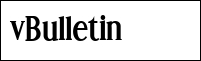atom0sFamily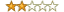Join Date: Jan 2015 Location: 127.0.0.1 Posts: 279 Rept. Given: 24 Rept. Rcvd 102 Times in 48 Posts Thanks Given: 46 Thanks Rcvd at 474 Times in 193 PostsCompiled with VS2013, the given name/key you posted does not work with this.
 The Following User Says Thank You to atom0s For This Useful Post: dila (07-19-2015)
#3
 QuakeGamerFriendJoin Date: Sep 2010 Posts: 53 Rept. Given: 3 Rept. Rcvd 8 Times in 6 Posts Thanks Given: 2 Thanks Rcvd at 38 Times in 20 PostsQuote:
 Originally Posted by atom0sCompiled with VS2013, the given name/key you posted does not work with this.
Compiled with g++ under linux. Serial worked for me.
 The Following User Says Thank You to QuakeGamer For This Useful Post: dila (07-19-2015)
#4
 dilaFriendJoin Date: Jan 2010 Posts: 60 Rept. Given: 12 Rept. Rcvd 32 Times in 14 Posts Thanks Given: 35 Thanks Rcvd at 74 Times in 20 Posts@atom0s I'm sorry, I have fixed the problem. The problem is the use of size_t as the return value on line 24 and not using uint64_t.

The full code tested on both Windows with MSVC 2013 and Linux with g++ is here, and now it accepts the original serial:

Code:
```/*
::::::::::::::::::::::
:: Keygenme by dila ::
::    July 2015     ::
::::::::::::::::::::::
*/

#include <iostream>
#include <stdint.h>
#include <string>

uint64_t rol(const uint64_t value, const int places) {
return (value<<places)|(value>>(64-places));
}

uint64_t ror(const uint64_t value, const int places) {
return (value>>places)|(value<<(64-places));
}

bool stringToInteger(const std::string& input, uint64_t* r) {
static const std::string lut = "0123456789abcdef";
if (input.length() == 16) {
*r = 0;
for (size_t i = 0; i < input.length(); ++i) {
uint64_t digit = lut.find_first_of(input[i]);
if (digit == std::string::npos) {
return false;
}
*r |= digit << (60 - i * 4);
}
return true;
}
return false;
}

uint64_t h0(const std::string& name) {
uint64_t r = ~0x3141592653589793ULL;
for (size_t k = 1; k <= 16; ++k) {
for (size_t i = 0; i < name.length(); ++i) {
int c = (13 + i * k) % 64;
r ^= rol(uint64_t(name[i]), c);
}
}
return r;
}

uint64_t h1(const uint64_t x) {
return x ^ ror(x, 13);
}

bool check(const std::string& name, const std::string& serial) {
uint64_t s;
if (stringToInteger(serial, &s)) {
if (h0(name) == h1(s)) {
return true;
}
}
return false;
}

int main() {
std::string name;
std::cout << "Name: ";
std::getline(std::cin, name);

std::string serial;
std::cout << "Serial: ";
std::getline(std::cin, serial);

if (check(name, serial)) {
std::cout << "Success! Thank you for registering." << std::endl;
} else {
std::cout << "Error. Invalid name/serial combination." << std::endl;
}

return 0;
}```
 The Following User Says Thank You to dila For This Useful Post: chessgod101 (07-20-2015)
#5
 ketanFriendJoin Date: Mar 2005 Posts: 101 Rept. Given: 0 Rept. Rcvd 12 Times in 5 Posts Thanks Given: 5 Thanks Rcvd at 84 Times in 48 Postsanother one is: 804DD0492B8B34C ;-)
 The Following User Says Thank You to ketan For This Useful Post: dila (07-25-2015)
#6
 arnixFriendJoin Date: Feb 2005 Posts: 68 Rept. Given: 11 Rept. Rcvd 18 Times in 7 Posts Thanks Given: 2 Thanks Rcvd at 6 Times in 4 PostsQuote:
 Originally Posted by dilaHi, I made a keygenme for you all to try. I am very interested to see how you solve it. Perhaps it is very easy for you!
Thanks for the keygenme, here is a dirty brute-force based keygen.
Attached Files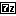kg.7z (1.1 KB, 4 views)

Last edited by arnix; 07-21-2015 at 03:57.
 The Following User Says Thank You to arnix For This Useful Post: dila (07-25-2015)
#7
 arnixFriendJoin Date: Feb 2005 Posts: 68 Rept. Given: 11 Rept. Rcvd 18 Times in 7 Posts Thanks Given: 2 Thanks Rcvd at 6 Times in 4 PostsThere was a small bug in the previous version. The keygenme wants keys with exactly 16 bytes long, so the smaller keys must be leaded with zeros (this is the reason why ketan's key won't be accepted by the keygenme (also must be in lowercase)). Here is a fixed version.
Attached Fileskg.7z (1.1 KB, 11 views)

Last edited by arnix; 07-21-2015 at 06:09.
 The Following User Gave Reputation+1 to arnix For This Useful Post: giv (07-21-2015)
 The Following 2 Users Say Thank You to arnix For This Useful Post: dila (07-25-2015), giv (07-21-2015)
#8
 ketanFriendJoin Date: Mar 2005 Posts: 101 Rept. Given: 0 Rept. Rcvd 12 Times in 5 Posts Thanks Given: 5 Thanks Rcvd at 84 Times in 48 Postshttp://pastebin.com/yQpTykRx
 The Following User Says Thank You to ketan For This Useful Post: dila (07-25-2015)
#9
 dilaFriendJoin Date: Jan 2010 Posts: 60 Rept. Given: 12 Rept. Rcvd 32 Times in 14 Posts Thanks Given: 35 Thanks Rcvd at 74 Times in 20 PostsThe objective was to take the input name and generate a working serial. Here is my analysis of the problem:

In general, a keygen must always be possible, since the software author has a means to generate working serials. And as I showed you, I had generated a key for the name "exetools", proving that I have a working keygen.

The core of the checking routine comes down to this comparison:

h0(name) = h1(serial)

A bt of algebra lets us transform this:

h1^-1(h0(name)) = h1^-1(h1(serial)

And the inverse functions cancel to give:

h1^-1(h0(name)) = serial

So to solve the keygenme is to find the inverse of the h1 function, then evaluate the above expression to produce a serial number from a given name.

I talked to João Marques on the Skype group about this (which you should join, incidentally), and he gave me his brilliant insight into the workings of the h1 function which allow you to solve it.

h1(x) := x ^ ror(x, 13)

He observed that this expression corresponds to a linear system of equations in the bits of x. Specifically, the following system:

x_0 + x_13 = y_0
x_1 + x_14 = y_1
x_2 + x_15 = y_2
: : :
x_n + x_([n+13]%k) = y_n

Now take a look at a simplified with a word length of 3 bits and rotate count of 1

x_0 + x_1 = y_0
x_1 + x_2 = y_1
x_2 + x_0 = y_2

The XOR behaves like carry-less addition modulo 2, and the system is completely determined once a single value has been chosen. So we start by setting any x_i to either 1 or 0, then all equations are solvable by back substitution.

Let x_0 = 0 then:

0 + x_1 = y_0
x_1 + x_2 = y_1
x_2 + 0 = y_2

implies that

x_1 = y_0
x_2 = y_2

Alternatively, let x_0 = 1, and arrive at the second solution to the system.

This is the solution implemented by ketan, requiring only word-length iterations to build up the solution key.

The h0() function can be implemented as-is and doesn't require any analysis.

So my question to you is this:

What is the smallest possible modification of the h1 function that will make the problem much harder, yet still possible, to solve.

If you start a new thread with your own problem of the form f(name) = g(serial), and I would be very interested in solving it with you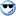The Following 2 Users Say Thank You to dila For This Useful Post: arnix (07-28-2015), foosaa (07-30-2015)

 Tags keygenmeThread ToolsShow Printable VersionEmail this Page Display ModesLinear ModeSwitch to Hybrid ModeSwitch to Threaded ModePosting Rules You may not post new threads You may not post replies You may not post attachments You may not edit your posts BB code is On Smilies are On [IMG] code is On HTML code is On Forum RulesSimilar Threads Thread Thread Starter Forum Replies Last Post sezar21m General Discussion 18 10-16-2013 01:19

All times are GMT +8. The time now is 07:58.

 Aaron's homepage - TopAlways Your Best Friend: Aaron, JMI, ahmadmansoor, ZeNiX
( 1998 - 2020 )